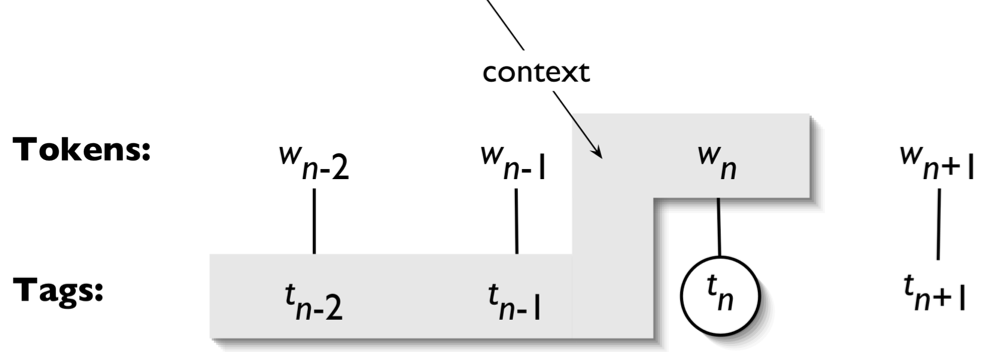# 自然语言处理 05：词性标注

## 墨尔本大学 COMP90042 课程笔记

Posted by YEY on March 17, 2020

# Lecture 05 词性标注

• 什么是词性（Part-of-Speech, POS）？
• 词性（POS） 又称 词类（word classes）、形态类别（morphological classes） 或者 句法类别（syntactic categories）
• 我们为什么关心词性？
因为词性在很大程度上告诉了我们一个词的句法功能以及它周围可能出现的词
• 名词（noun）通常出现在限定词（determiner，例如：$\textit{the}$、$\textit{a}$ ）后面。
• 动词（verb）通常出现在名词（noun）后面。
• 并且，一个词的发音可能会根据词性不同而改变。
例如：对于 $\textit{content}$ 这个单词，当其作为名词（noun）时，重音在前，读作 $\textit{CONtent}$；而当其作为形容词（adjective）时，重音在后，读作 $\textit{conTENT}$。

• 训练数据：
• $\textit{“The lawyer convinced the jury.”}\to \textit{Sam}$
• $\textit{“Ruby travelled around Australia.”}\to \textit{Sam}$
• $\textit{“The hospital was cleaned by the janitor.”}\to \textit{Max}$
• $\textit{“Lunch was served at 12pm.”}\to \textit{Max}$

这里，我们有 $4$ 个句子，前两个作者是 $\textit{Sam}$，后两个来自 $\textit{Max}$。通过观察可以发现他们在使用句子时存在不同的模式，$\textit{Sam}$ 倾向于使用 主动语态（active voice），$\textit{Max}$ 倾向于使用 被动语态（passive voice）

• 测试数据：
$\textit{“The bookstore was opened by the manager.”}\to \text{?}$
• 我们可以很容易判断它很可能来自 $\textit{Max}$，因为它们具有相似的 结构（被动语态）
• 作为人类，我们可以很容易捕获这种句子模式。
• 但是，简单的 词袋表示（BOW representation） 无法捕获这种句子结构层面的差异。因为在词袋模型中，句子单词之间的顺序信息被直接丢弃掉了。
• 如何确保计算机能够知道/学习这种结构层面的信息？词性信息可能会有帮助。
• 信息提取（Information Extraction）
另一个涉及单词词性的场景是信息提取，其目的是试图从语料库中提取到关系信息。
• 给定如下的语料库：
• $\textit{“Brasilia, the Brazilian capital, was founded in 1960.”}$
• 我们希望从上面的句子中提取某些关系：
• $\textit{capital (Brazil, Brasilia)}$
• $\textit{founded (Brasilia, 1960)}$
• 这其中涉及到很多步骤，但是首先我们需要知道词性信息，例如：
• 名词：$\textit{Brasilia, capital}$
• 形容词：$\textit{Brazilian}$
• 动词：$\textit{founded}$
• 数字：$\textit{1960}$

• 词性
• 标注集
• 自动标注

## 1. 词性

### 1.2 开放类（英语）

• 名词（Nouns）
• 专有名词（proper noun）vs. 一般名词（common noun）
例如：$\textit{Australia}$ （澳大利亚） vs. $\textit{wombat}$ （毛鼻袋熊）
• 不可数名词（mass noun）vs. 可数名词（count noun）
例如：$\textit{rice}$ （大米） vs. $\textit{bowls}$ （碗）
• 动词（Verbs）
• 丰富的形态变化（rich inflection）
例如：$\textit{go/goes/going/gone/went}$
• 助动词（auxiliary verbs）
例如：英语中的 $\textit{be, have, do}$
• 及物性（transitivity）
后面可以带不同数量的参数。 例如：$\textit{wait }$ vs. $\textit{hit }$ vs. $\textit{give}$
$\textit{Alice waits.}$
$\textit{Alice hits Bob.}$
$\textit{Alice gives Bob a book.}$
例如：$\textit{happy}\to \textit{a bit happy, very happy, not very happy}$
例如：$\textit{computational}\quad$ vs. $\quad\textit{noncomputational}$
• 表方式（manner）
例如：$\textit{slowly}$
• 表位置（locative）
例如：$\textit{here}$
• 表程度（degree）
例如：$\textit{really}$
• 表时态（temporal）
例如：$\textit{yesterday}$
注意，$\textit{yesterday}$ 是一个很有趣的例子，它的词性可以是名词或者副词，具体情况取决于其在句子中扮演的角色。

### 1.3 封闭类（英语）

• 介词（Prepositions）
通常用来在两个事物之间建立某种联系。
• $\textit{in, on, with, for, of, over, \dots}$
例如：$\textit{an apple on the table}$
• 小品词（Particles）
通常是介词里的一些单词，但是用法上存在一些区别，经常用在动词后面，构成一些词组。
• 例如：$\textit{brushed himself off}$
从表意上看，$\textit{brush}$（刷） 和 $\textit{brush off}$（刷掉） 有一些差别。在英语中，小品词与动词一起使用通常代表了一些约定俗成的规则用法。
• 限定词（Determiners）
• 冠词（articles）
例如：$\textit{a, an, the}$
• 指示代词（Demonstratives）
例如：$\textit{this, that, these, those}$
• 量词（Quantifiers）
例如：$\textit{each, every, some, two, \dots}$
• 代词（Pronouns）
• 人称（Personal）
例如：$\textit{I, me, she, \dots}$
• 所有格（Possessive）
例如：$\textit{my, our, \dots}$
• 疑问（Interrogative 或者 $\textit{Wh-}$）
例如：$\textit{who, what, \dots}$
• 连接词（Conjunctions）
连接两个分句（clauses）。
• 并列（Coordinating）
例如：$\textit{and, or, but}$
• 从属（Subordinating）
例如：$\textit{if, although, that, \dots}$
• 情态动词（Modal verbs）
• 表能力（Ability）
例如：$\textit{can, could}$
• 表许可（Permission）
例如：$\textit{can, may}$
• 表可能性（Possibility）
例如：$\textit{may, might, could, will}$
• 表必要性（Necessity）
例如：$\textit{must}$
• 还有很多其他词性
• 否定（negatives）、礼貌标记语（politeness markers）等等。

### 1.4 模糊性

• 让我们来看一个例子，请比较下面两个句子：
• $\textit{Time flies like an arrow}$ （光阴似箭）
• $\textit{Fruit flies like a banana}$ （果蝇喜欢香蕉）
$\textit{Time}$ $\textit{flies}$ $\textit{like}$ $\textit{an}$ $\textit{arrow}$
noun verb preposition determiner noun

$\textit{Fruit}$ $\textit{flies}$ $\textit{like}$ $\textit{a}$ $\textit{banana}$
noun noun verb determiner noun

• 接下来，让我们来看一下新闻标题中的词性模糊性：
• $\textit{British Left Waffles on Falkland Islands}$
第一眼单纯从字面意思上来看，我们可能会理解为：
[$\textit{British}$] [$\textit{Left}$] [$\textit{Waffles}$] [$\textit{on}$] [$\textit{Falkland Islands}$]
英国人在福克兰群岛上留下了一些华夫饼
但是如果你是英国人，你就会知道 “$\textit{waffle}$” 除了 “华夫饼” 之外，还可以表示 “尚未决定” 的含义，同时 “$\textit{left}$” 除了 “留下来” 之外，还有 “左派” 的含义。所以，结合语境，这个新闻标题实际想表达的含义是：
[$\textit{British Left}$] [$\textit{Waffles}$] [$\textit{on}$] [$\textit{Falkland Islands}$]
英国左派党尚未确定（对于）福克兰群岛（的政策）

• $\textit{Juvenile Court to Try Shooting Defendant}$
同样，第一眼看标题，我们可能会理解为：
[$\textit{Juvenile Court}$] [$\textit{to}$] [$\textit{Try}$] [$\textit{Shooting}$] [$\textit{Defendant}$]
少年法庭试图射杀被告
但事实上，这里唯一的动词只有 “$\textit{try}$”，它除了 “尝试” 之外，还有 “审判” 的意思。而后面的 “$\textit{shooting defendant}$” 整体作为一个宾语，表示 “枪击案被告”。所以，这个标题实际想表达的含义是：
[$\textit{Juvenile Court}$] [$\textit{to}$] [$\textit{Try}$] [$\textit{Shooting Defendant}$]
少年法庭将审判枪击案被告

• $\textit{Teachers Strike Idle Kids}$
同样，第一眼看标题，我们可能会理解为：
[$\textit{Teachers}$] [$\textit{Strike}$] [$\textit{Idle}$] [$\textit{Kids}$]
教师殴打懒散的孩子
这在过去可能还能够理解，但是如今这个句子实际上表达的是另外一层意思。“$\textit{Strike}$” 除了 “殴打”，还有 “罢工” 的意思。实际上，句子中并不存在动词。 “$\textit{Teachers strike}$” 整体作为原因，后面的 “$\textit{idle kids}$” 整体作为结果：
[$\textit{Teachers Strike}$] [$\textit{Idle Kids}$]
教师罢工（导致）孩子们比较闲散

• $\textit{Eye Drops Off Shelf}$
同样，第一眼看标题，我们可能会理解为：
[$\textit{Eye}$] [$\textit{Drops}$] [$\textit{Off}$] [$\textit{Shelf}$]
眼睛从架子上掉下来了
但显然，这种解释并不符合常理。“$\textit{Eye drops}$” 在这里作为一个整体，表示 “滴眼液”。而 “$\textit{off shelf}$” 也作为一个整体，表示 “下架”。所以句子实际意思为：
[$\textit{Eye Drops}$] [$\textit{Off Shelf}$]
滴眼液下架了

## 2. 标注集

• 作为词性（POS）信息的一种紧凑表示：
• 通常长度 $\le 4$ 个大写字母
• 一个大类下面可能包含好几个子类：通常包含对于形态变化的区分。
• 几种主要英语标注集：
• Brown (87 tags)
• Penn Treebank (45 tags)
• CLAWS/BNC (61 tags)
• “Universal” (12 tags)

最后一种 “Universal Treebank” 之所以只有 12 种 tags 是因为该标注集是基于一个跨语言标注的项目：它试图发现一些独立于语言之外的结构和关系，所以它只涵盖了 12 种适用于大部分语言的 tags。

• 对于所有的主流语言，都至少有一个标注集。

### 2.1 主要的 Penn Treebank Tags

Tag 词性 Tag 词性
NN noun（名词） VB verb（动词）
DT determiner（限定词） CD cardinal number（基数词）
IN preposition（介词） PRP personal pronoun（人称代词）
MD modal（情态动词） CC coordinating conjunction（并列连词）
RP particle（小品词） WH wh-pronoun（wh 代词）
TO $\textit{to}$

### 2.2 Penn Treebank 的衍生 Tags

• NN（名词）
• NNS（plural，复数）：$\textit{wombats}$
• NNP（proper，专有）：$\textit{Australia}$
• NNPS（proper plural，专有复数）：$\textit{Australians}$
• VB（动词）
• VB（infinitive，不定式）：$\textit{eat}$
• VBP（1st / 2nd person present，第一、第二人称）：$\textit{eat}$
• VBZ（3rd person singular，第三人称单数）：$\textit{eats}$
• VBD（past tense，过去时）：$\textit{ate}$
• VBG（gerund，动名词）：$\textit{eating}$
• VBN（past participle，过去分词）：$\textit{eaten}$
• JJ（形容词）
• JJR（comparative，比较级）：$\textit{nicer}$
• JJS（superlative，最高级）：$\textit{nicest}$
• RB（副词）
• RBR（comparative，比较级）：$\textit{faster}$
• RBS（superlative，最高级）：$\textit{fastest}$
• PRP（人称代词）
• PRP（possessive，所有格）：$\textit{my}$
• WH（疑问代词）
• WH（possessive，所有格）：$\textit{whose}$
• WDT（wh-determiner，wh 限定词）：$\textit{who}$
• WRB（wh-adverb，wh 副词）：$\textit{where}$

### 2.3 标注文本例子

$\textit{The}$/DT $\textit{limits}$/NNS $\textit{to}$/TO $\textit{legal}$/JJ $\textit{absurdity}$/NN $\textit{stretched}$/VBD $\textit{another}$/DT $\textit{notch}$/NN $\textit{this}$/DT $\textit{week}$/NN $\textit{when}$/WRB $\textit{the}$/DT $\textit{Supreme}$/NNP $\textit{Court}$/NNP $\textit{refused}$/VBD $\textit{to}$/TO $\textit{hear}$/VB $\textit{an}$/DT $\textit{appeal}$/VB $\textit{from}$/IN $\textit{a}$/DT $\textit{case}$/NN $\textit{that}$/WDT $\textit{says}$/VBZ $\textit{corporate}$/JJ $\textit{defendants}$/NNS $\textit{must}$/MD $\textit{pay}$/VB $\textit{damages}$/NNS $\textit{even}$/RB $\textit{after}$/IN $\textit{proving}$/VBG $\textit{that}$/IN $\textit{they}$/PRP $\textit{could}$/MD $\textit{not}$/RB $\textit{possibly}$/RB $\textit{have}$/VB $\textit{caused}$/VBN $\textit{the}$/DT $\textit{harm}$/NN ./.

## 3. 自动标注

• 对于形态学分析（morphological analysis）非常重要。
• 例如：词形还原（lemmatisation）。
• 对于某些应用，我们希望关注某些特定的 POS。
• 例如：名词对于信息提取非常重要，而形容词对于情感分析非常重要。 所以在处理某些任务时，需要思考词性标注如何能够潜在地帮助我们更好地完成任务。
• 对于特定的分类任务可能是非常有用的特征。
• 例如：流派分类（genre classification）。
• POS 可以帮助单词消除歧义（disambiguation）
• 例如：$\textit{cross}$/NN $\quad$ vs. $\quad$ $\textit{cross}$/VB $\quad$ vs. $\quad$ $\textit{cross}$/JJ
• 可以利用它们创建更大的结构（句法分析）

• 基于规则的标注器（Rule-based taggers）
• 统计标注器（Statistical taggers）
• Unigram 标注器、biigram 标注器等等
• 基于分类器的标注器（Classifier-based taggers）
尤其是对于文本分类任务，输入是一个单词，以及一些其他特征（例如：相邻单词），类别标签对应不同的 POS，然后训练分类器，对单词进行 POS 分类。
• 隐马尔可夫模型标注器（Hidden Markov Model, HMM, taggers）
这种方法涉及一些关于 马尔可夫链 的假设，我们将在下节课对其进行较为详细的讨论。

### 3.1 基于规则的标注器

• 通常从每个单词的可能标签列表（a list of possible tags）开始
• 来自一个词汇资源或者语料库
• 通常包含一些其他的词汇信息
• 例如：动词的次类（subcategorisation），即参数（arguments）
• 应用规则将范围缩小到单个 tag
• 例如：如果单词前面是 DT（限定词），那么我们可以排除 VB（动词）的可能性
• 依赖一些清晰的上下文信息（unambiguous contexts）
• 基于规则的标注系统会非常庞大，因为可能存在上千种规则约束。虽然这种方法的精度很高，但是其扩展性较差，它并不能很好地适应新的语言，例如：如今随着互联网的发展，人们发明了很多网络语言。

### 3.2 Unigram 标注器

Unigram 标注器是一种非常简单的标注器。

• 对于每个单词 type 分配最常见的 tag
• 需要一个已经进行过词性标注的语料库
• “模型”只是一个查询表（a look-up table）
• 但是准确率（accuracy）很高，能达到 $90\%$ 左右
• 能够正确处理约 $75\%$ 左右的歧义（ambiguity）
• 通常作为一些更加复杂的方法的 baseline

### 3.3 基于分类器的标注器

• 训练一个标准的判别式（discriminative）分类器（例如：logistic 回归、神经网络等等），其输入特征为：
• 目标单词
• 单词附近的词汇上下文
• 句子中已经完成过分类的 tags例如，在上面的例子中，我们是按照从左至右的顺序进行标注的，所以当我们对单词 $w_n$ 进行标注时，我们已经得到了它前面两个单词 $w_{n-2}, w_{n-1}$ 的词性，即 $t_{n-2}, t_{n-1}$，因此，我们可以在分类时利用这些已知信息。

• 最好的序列标注模型之一
• 但是会碰到 错误传播（error propagation） 的问题：先前步骤中的错误预测会影响后面单词的词性预测。这种错误只会发生在对测试数据进行预测时，因为在训练过程中，我们用到的是已经正确标注的信息，而在预测过程中，我们会依赖之前的预测结果。对于基于分类器的方法而言，这是一个很重要的问题，有很多对应的处理办法。

### 3.4 隐马尔可夫模型

• 一种基本的序列（或者结构）模型
• 类似序列分类器，HMM 也利用了之前的 tag 和 lexical evidence
• 不同于序列分类器的是，HMM 假设之前的 tag evidence 和 lexical evidence 之间完全相互独立，这样可以带来如下好处：
• 更小的稀疏性（sparsity）
• 对于序列预测（即找到整个单词序列的最佳 tagging）而言，是一种非常快速的算法，因为可以利用维特比译码（Viterbi decoding）来找到整个单词序列的最佳序列标注，而这在前面的分类器方法中很难实现。

### 3.5 未知单词

• 在形态丰富的语言（morphologically rich languages）中，未知单词是一个巨大的问题。
例如：土耳其语（Turkish）
• 可以利用我们仅见过一次的罕用词（hapax legomena，即出现频率只有一次的单词）来最好地猜测我们从未见过的单词。
• 倾向于是名词，之后是动词
• 不太可能是限定词
• 可以利用子词（sub-word）表示来捕获形态学特征（寻找常见词缀）

## 4. 总结

• 词性（Part of speech）是语言学和自动文本分析之间的一个基本交汇点。
• NLP 中的一项基本任务，可以为许多其他应用程序提供有用的信息。
• 应用于词性标注的方法通常是语言学任务中的典型代表，例如：概率方法、序列机器学习

## 5. 扩展阅读

• JM3 Ch. 8 8.1-8.3, 8.5.1本作品采用知识共享署名-非商业性使用-相同方式共享 4.0 国际许可协议进行许可。 欢迎转载，并请注明来自：YEY 的博客 同时保持文章内容的完整和以上声明信息！